• Introduction
• What is in this manual
• What is Caspoc
• User interface
• Introduction
• Starting
• Simulation
• Editing
• Viewing and printing
• Getting Started
• Basic editing
• Simulation in the time domain
• Basic User Interface Topics
• Editing
• Simulation
• Viewing
• Library
• Reports
• Project management
• Circuit and Block Diagram Components
• Introduction
• Cscript and user defined functions
• Component parameters
• Modeling Topics
• Introduction
• Power Electronics
• Semiconductors
• Electrical Machines
• Electrical drives
• Power Systems
• Mechanical Systems
• Thermal Systems
• Magnetic Circuits
• Green Energy
• Coupling to FEM
• Experimenter
• Analog hardware description language
• Embedded C code Export
• Coupling to Spice
• Small Signal Analysis
• Matlab coupling
• Tips and tricks
• Appendices

## Reduced Order Modeling thermal systems.

In this tutorial the use of the loss predicting models connected to Reduced Order Models for heat sinks is explained. There are basically two thinks that have to be taken care of; modeling the losses in the power electronics circuit and the reduced order modeling of the heat sink in Ansys by the MOR method.

The first part discusses the power electronics model for fast loss prediction, the second part discusses the coupling to the MOR model.

Power electronics modeling

Basically all non-linear semiconductor models can be coupled to a heat sink. Only the basic switching models without switching loss determination have no thermal connection. Most of the semiconductor models found in the Components/Library/Semiconductor section have a thermal connection. This connection models the thermal and power flow via one connection. The power flow is through the connection, while the temperature is measured relative to another thermal connection. A comparison is with an electrical node, where the power flow equals the current and the temperature equals the voltage. All thermal models are based on this connection and in that way the MOR models can be coupled with the other thermal models.

In this tutorial only the fast loss prediction semiconductor models are discussed. You can select such a module from components/library/semiconductor/IGBT and from components/library/semiconductor/Mosfet.

These models are based on the ideal switch model and their losses are predicted based on data sheet variables. Since there is no non-linear model required for predicting the losses (they come from the data sheet values), the simulation can be relatively fast. If also the switching characteristic has to be simulated like gate charging and the delay in turning on and off, the IGBT and Mosfet models have to be used. They can be found in the same folder. All parameters for the modules can be found in the data sheet provided by the manufacturer. Modeled are on state losses, and switching losses, both temperature depending. Both the IGBT Module and Mosfet Module model are nearly equal, only the Mosfet model has no on state voltage drop.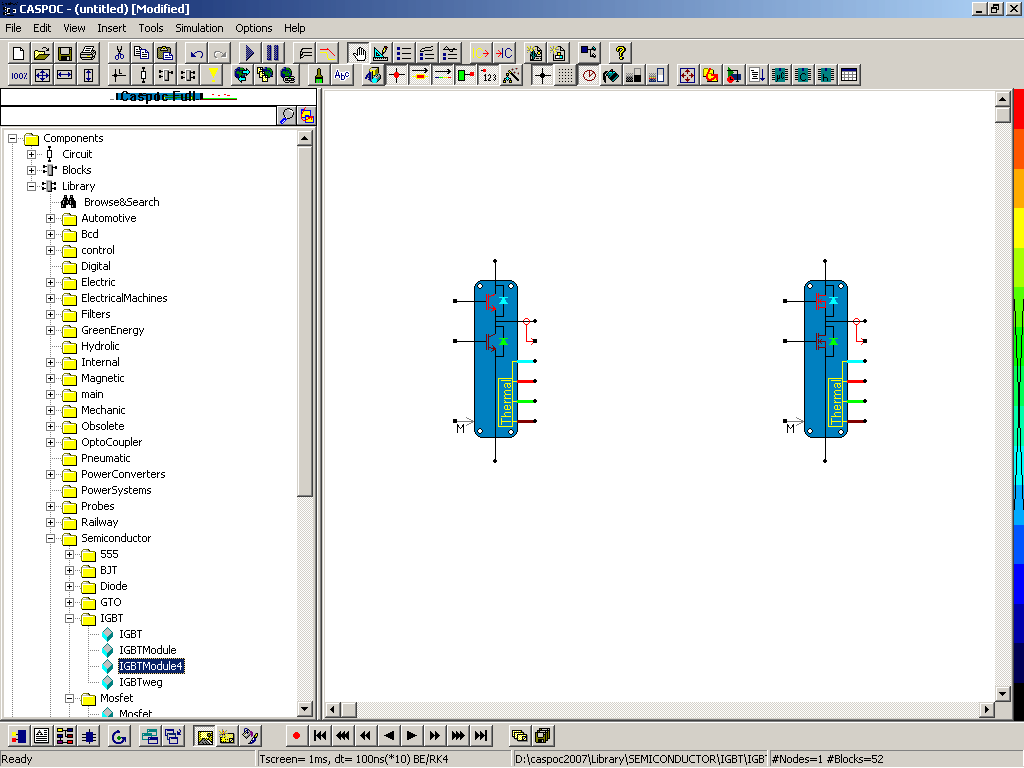You select such a model by clicking and releasing the left mouse button over the module name IGBTModule4. Move the mouse over the work screen and place the component by clicking the left mouse button again. You can move the component by clicking it with the left mouse button, hold the button down and move the component. Releasing the left mouse button places the component. To draw wires, simply click with the left mouse button on one of the inputs of the components, drag the mouse, while holding the left mouse button down and finalize the drawing by releasing the left mouse button. (More detail editing details can be found in the first chapters of the users-guide.)

The module shows a number of pins for connecting the component in a circuit. The electrical connections are visible from the icon on the component. The Collector, Emitter, Drain, Source as well as the Gate connection can be connected directly in an electric circuit. The connection on the left bottom side, with the arrow and M, is for connecting a model name block through the block diagram. The connection below the middle point with the red circle and red arrow are for measuring the power losses and other internal variables in the module. Both the model and measurement connection have a square node and they connection is optional.

The Gate nodes are also block diagram nodes and have to be connected to the controlling blocks. The signal can have a simple 0-1 value to control the IGBT or Mosfet. Remember that we re here modeling fast loss prediction models based on the switch model.

Note that all other nodes are round dots, meaning circuit nodes with through and cross variables, while block diagram nodes are square dots that represent only a numerical value.

Semiconductor Parameters

The parameters for the semiconductors are defined in the properties dialog box that can be opened by clicking the component with the right mouse button. Here the electrical parameters are defined for both 25 and 125 degrees. The parameters have to be specified for the IGBT/Mosfet and for the Diode.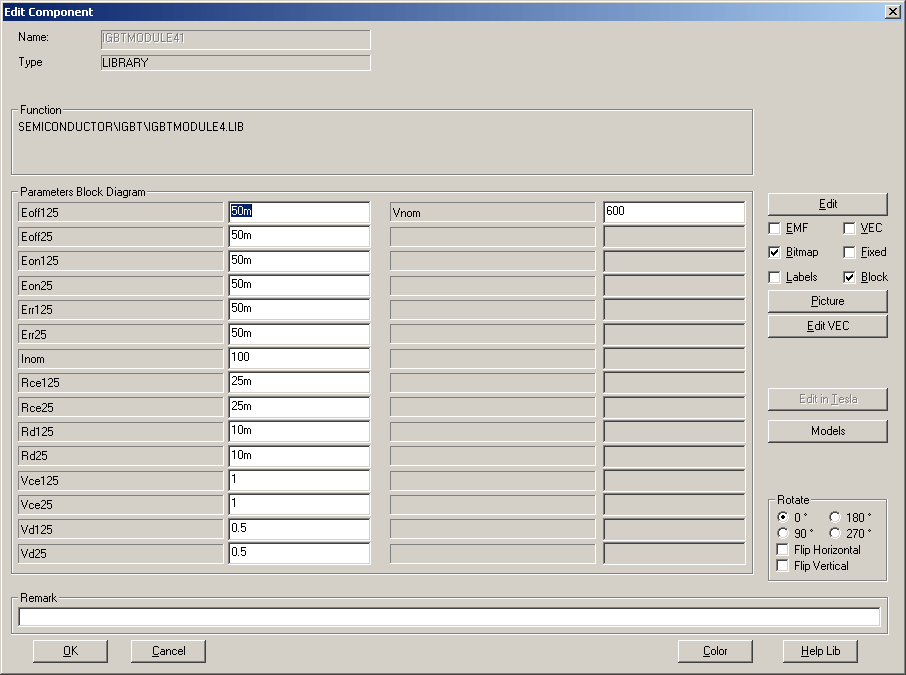The parameters Eon and Eoff specify the switching loss of the IGBT/Mosfet for each switching state. In the data sheet they are specified at a nominal voltage and current. For example an IGBT that is designed to operate at bus voltage of 600Volt, the parameters Eon and Eoff are specified at 600Volt. Also the nominal current is specified. In this modules the switching losses are dependent on the voltage across and the current through the semiconductor and the switching loss is scaled linearly. The scaling is also linearly dependent on the temperature. Notice that Eon and Eoff have to be specified for the correct value of the gating resistance. Therefore the data sheet provides different values of Eon and Eoff depending on Rgate.

The parameter Err defines the reverse recovery losses of the freewheeling diode. The turn-on losses for the diode are negligible, since the diode turns on soft.

All the switching losses are defined for the nominal voltage and current specified at Inom and Vnom.

The on state losses are defined by the on state voltage drop of the semiconductor and the resistive losses due to the slope in the on characteristic of the semiconductor. This is modeled by a series connection of the on state forward voltage drop and a series resistor. Both the on state voltage drop and resistor have to be specified for the 25 and 125 degrees Celsius. The diode parameters are Vd*25 and Rd*25, the IGBT parameters are Vce*25 and Rce*25,while the Mosfet parameters are denoted by Vds*25 and Rds*25. Here the * should be replaced by 1 for the parameter for 125 degrees Celsius, or omitted for the parameter for 25 Degrees Celsius.

The parameter can also be supplied from a .Model parameter data base, via the M connection on the module . More information on using the .Model connection is found in the user guide in the section on model parameters. (Block Mname and .Model)

Thermal connections

The four connections on the right bottom side are the thermal connections. These connections have to be connected to a thermal model or to the MOR model. Since in this module there are four components that can be specified independently, there are also four thermal connections. Since these are thermal circuit nodes, you can combine them to one node and connect them to a single thermal model.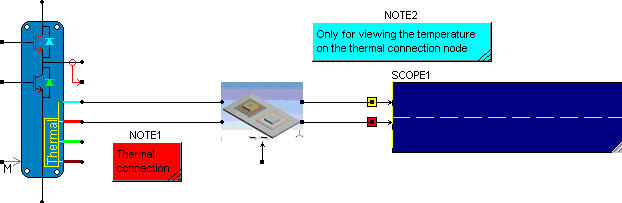In the above figure the two out of four thermal nodes are connected to a thermal model that has two thermal nodes. The thermal nodes are colored to identify them to their corresponding semiconductor. Light blue and red are the connections for the high side of the module, while green and brown represent the low side of the module.

The thermal model used in this tutorial is MOR2 since it has two thermal connections. The connections on the right side of the MOR model shown above are only for viewing the temperature on the thermal nodes and have no physical connections, nor are they required in the modeling process.

MOR coupling

The MOR model is a Reduced Order Model that is directly calculated form the Finite Element Matrix in Ansys using the MOR method. Therefore it incorporates the complete thermal model set up in Ansys and no external models are required. Only one parameter, the ambient temperature and the corresponding Reduced Order Model name have to be defined. The ambient temperature is defined in the properties dialog box for the MOR model. The Reduced Order Model is specified using the connection at the bottom of the MOR model.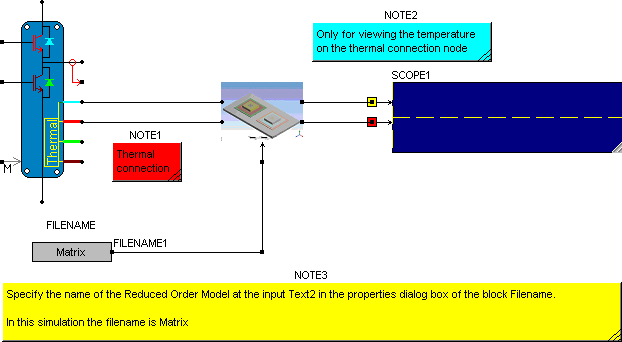The block Filename is found in components/blocks/miscellaneous. Specify the file name of the reduced order model at the input field Text2 in the properties dialog box. In this case the filename is Matrix.

From Ansys 4 data files are generated; Matrix.A, Matrix.B, Matrix.C and Matrix.E. Since their file name is equal and only the extension differs, only the file name (without extension) has to be specified. The thermal nodes from the MOR model have to be connected to a semiconductor thermal connection. The connections on the right side are only used to view the temperature in a scope, without drawing excessive wires in your schematic. You could have also connected the scope directly to the thermal nodes to view the temperature.

To show the correctness of the model, the following example compares the simulation results from a MOR model with the results from a thermal transient analysis in Ansys. In Ansys the temperature is stored in a text file during a thermal transient simulation. In Caspoc we set up two models. The first model is the MOR model connected to two power sources. The second model shows two look-up tables that give the temperature. Since in Ansys a transient simulation was performed, the look-up table has the temperature stored as function of time. Therefore the input of the look-up tables is the output from the block Time that gives the running simulation time in seconds.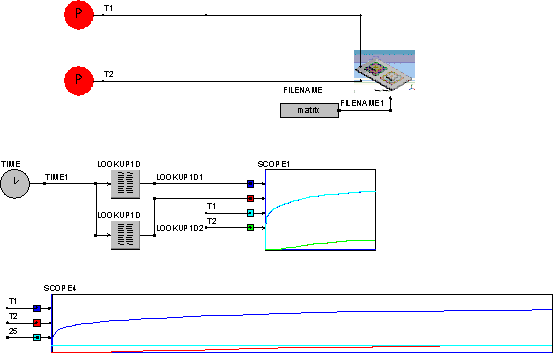The outputs are compared in scope 1 and you can see that both results from the transient analysis in Ansys and the MOR model agree very well. The bottom scope shows the temperature rise if one of the thermal nodes (node 1) is supplied with power. The temperature on node 1 is rising fast, while the temperature on node 2 is slowly rising due to the internal heat transport in the modeled structure.

Inverter simulation

Putting it all together, the switching losses from the fast loss predicting models can be fed into thermal MOR model. The advantage is that the simulation of the fast loss predicting models is much faster that using detailed non-linear models and the thermal MOR model is an accurate thermal model for any three-dimensional structure.

The example simulation is a simple single-phase full bridge with RL load. A gate control block creates the PWM signals, where the switching frequency and the delay between high side and low side control signal can be specified. Two IGBT modules are used and connected to four thermal MOR models. Each thermal MOR model has two thermal connections. The losses of the IGBT Modules P1 and P2 are measured from the red connection on the IGBT module and are for viewing purposes only. They are displayed in the lower scope. The current through and the voltage across the RL link is shown in the upper scopes.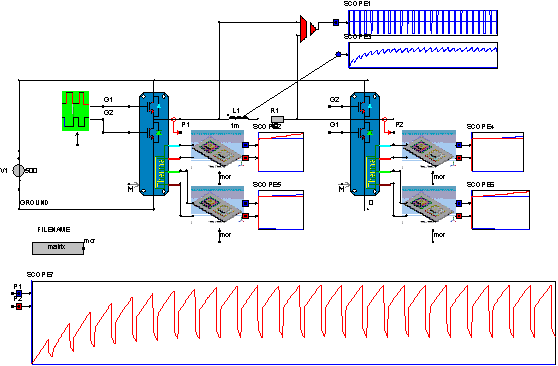The temperature of the semiconductors is displayed in the scopes on the right side of the MOR thermal models. From the temperature rise it is visible that in this case the High side from the left module and the Low side form the right module are producing more heat. The heat produced on the Low side in the left module and the High side in the right module is from the freewheeling diodes.

The file name of the Reduced Order Model from Ansys is "Matrix". This file name is specified in the block Filename. The output form this block is labeled "mor". The bottom connections of the thermal MOR models is also labeled "mor". Therefore the block Filename is directly coupled to all four MOR models and all four MOR models are based on the Reduced Order Model called "Matrix".

To customize this simulation, one can create new Reduced Order Models and store them under a new file name, for example "Matrix2". All that has to be done is the change the name "Matrix" into "Matrix2" in the block Filename and the simulation can be repeated for the new structure.

The parameters for the semiconductors are default parameters. They have to be set according to the data sheet values.

Coupling the MOR model with non-linear thermal models.

The MOR is essentially a linear model. However there are many thermal models being non-linear, such as radiation. In Caspoc the radiation model can be coupled to the MOR model. In fact, since the MOR model is a thermal model with thermal nodes, several MOR models can be mixed, also with standard thermal models from the Caspoc library. The MOR model is interfacing with the other thermal models through its thermal nodes. Since it does not mater if such a node is connected to a heat source or heat sink, the MOR model can freely be connected to other thermal models.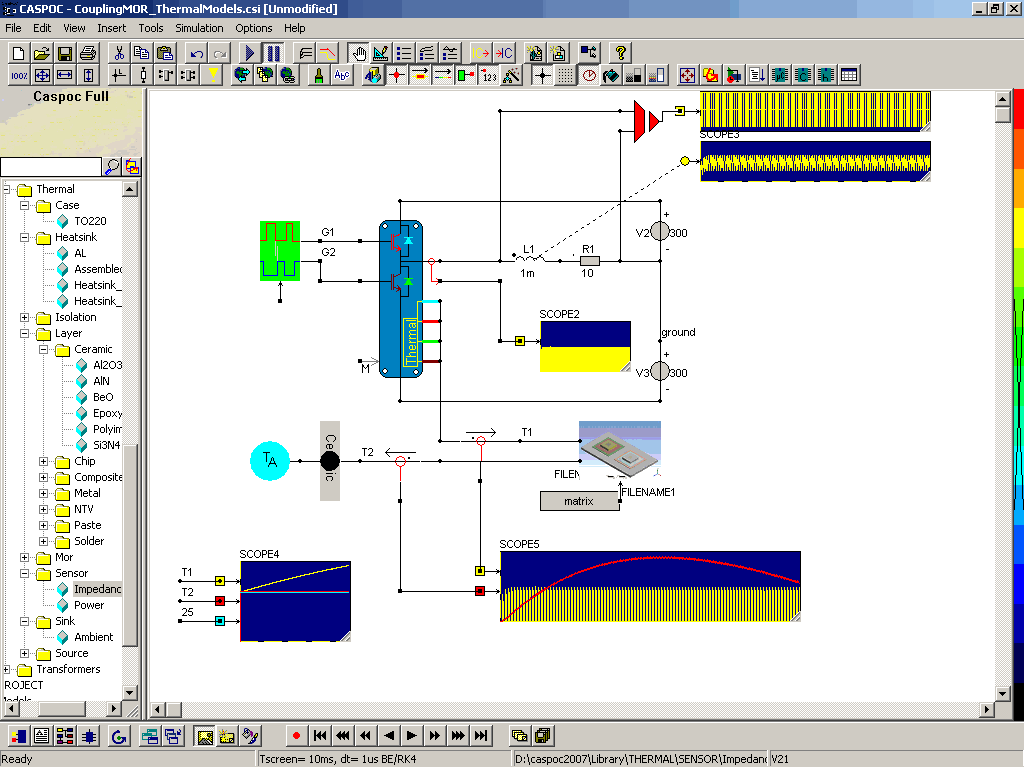In the above simulation the losses go through the MOR model and one Ceramic layer. As can be seen in scope 5, the losses gets somehow filtered to a more continuous power flow. In this way non-linear thermal models can be mixed with MOR models.